# Static Analysis u Automatic derivation of static properties

• Slides: 51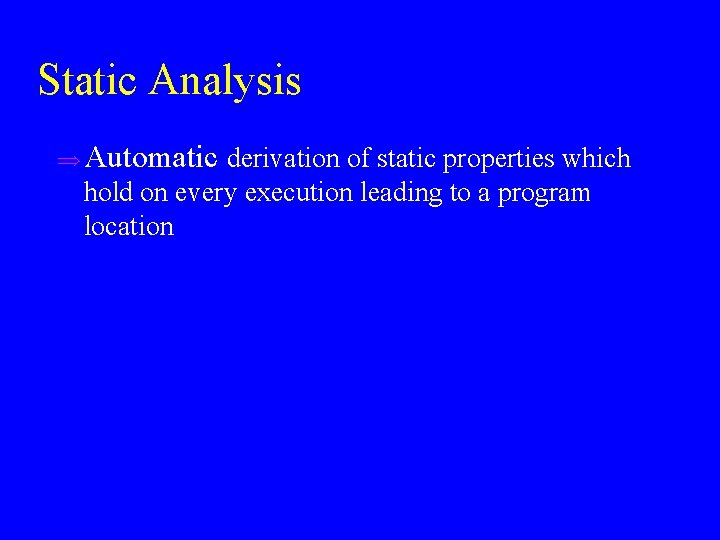Static Analysis u Automatic derivation of static properties which hold on every execution leading to a program locationExample Static Analysis Problem u Find variables with constant value at a given program location u Example program int p(int x){ return x *x ; void main()} { int z; if (getc()) z = p(6) + 8; else z = p(-7) -5; printf (z); } 44Recursive Program int x void p(a) { read (c); if c > 0 { a = a -2; p(a); a = a + 2; } x = -2 * a + 5; print (x); } void main { p(7); print(x); }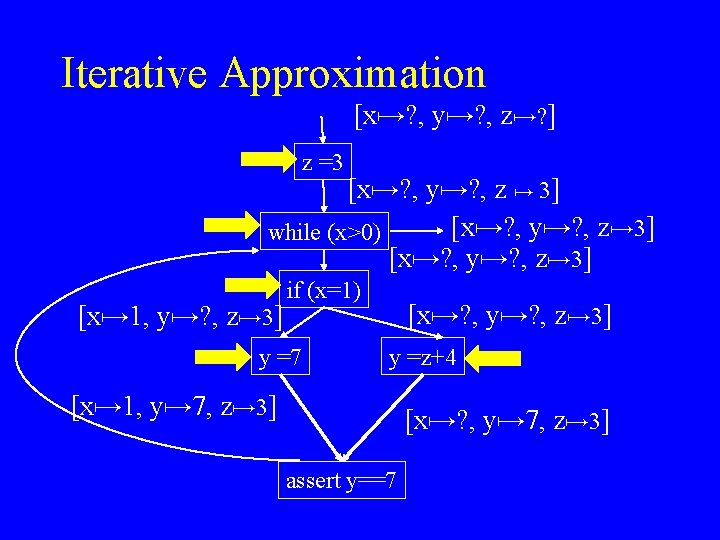Iterative Approximation [x ? , y ? , z ? ] z =3 [x ? , y ? , z 3] [x ? , y ? , z 3] while (x>0) [x ? , y ? , z 3] [x 1, y ? , z 3] if (x=1) y =7 [x ? , y ? , z 3] y =z+4 [x 1, y 7, z 3] [x ? , y 7, z 3] assert y==7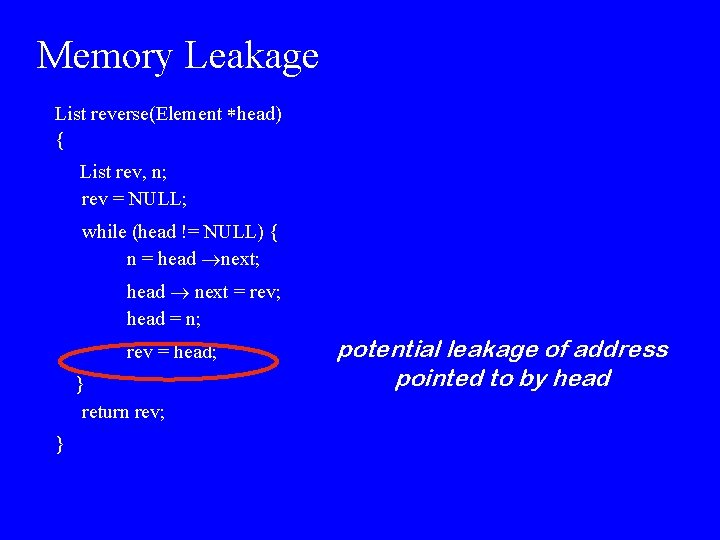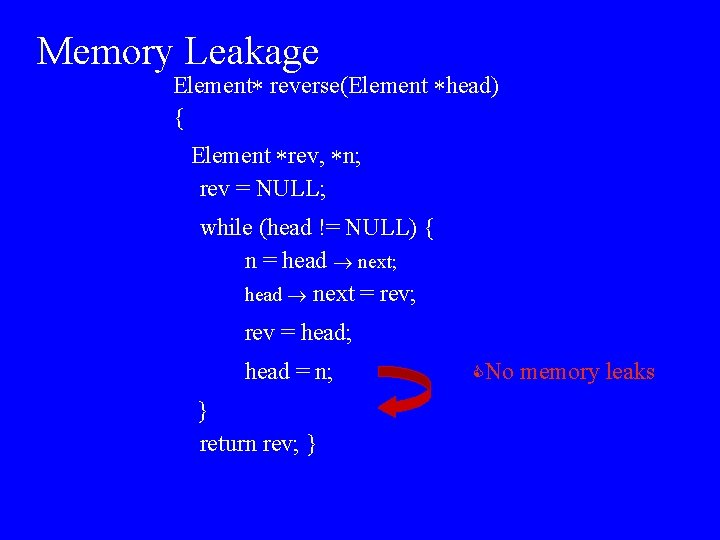Memory Leakage Element reverse(Element head) { Element rev, n; rev = NULL; while (head != NULL) { n = head next; head next = rev; rev = head; head = n; } return rev; } CNo memory leaks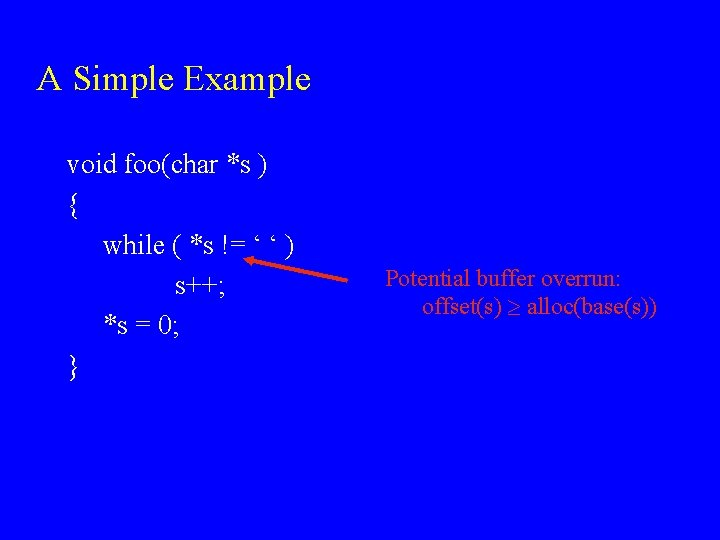A Simple Example void foo(char *s ) { while ( *s != ‘ ‘ ) s++; *s = 0; } Potential buffer overrun: offset(s) alloc(base(s))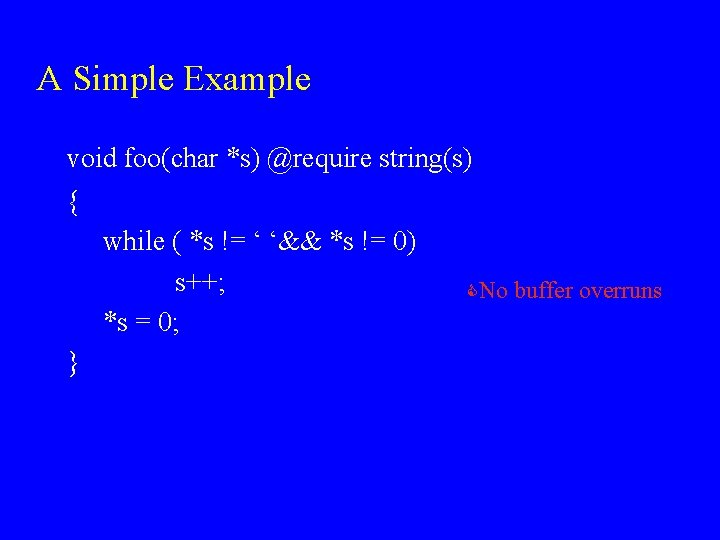A Simple Example void foo(char *s) @require string(s) { while ( *s != ‘ ‘&& *s != 0) s++; *s = 0; } CNo buffer overrunsExample Static Analysis Problem u Find variables which are live at a given program location u Used before set on some execution paths from the current program point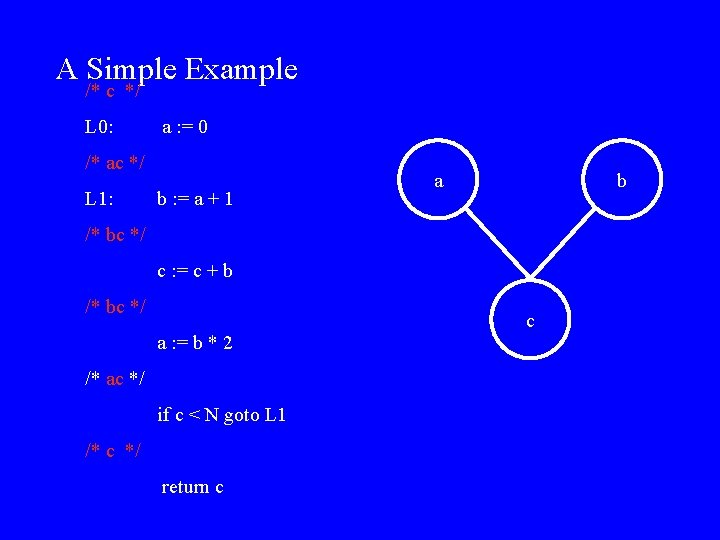A Simple Example /* c */ L 0: a : = 0 /* ac */ L 1: b : = a + 1 a b /* bc */ c : = c + b /* bc */ c a : = b * 2 /* ac */ if c < N goto L 1 /* c */ return c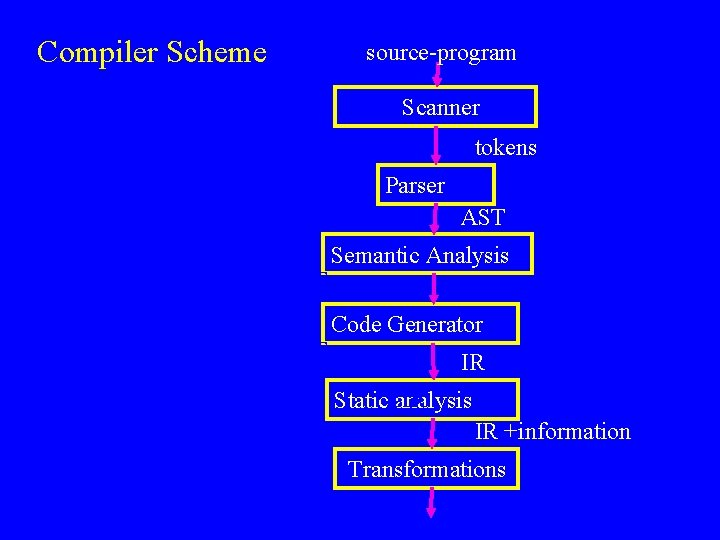Compiler Scheme source-program String Tokens AST Scanner tokens Parser AST Semantic Analysis Code Generator IR Static. LIR analysis IR +information TransformationsOther Example Program Analyses u u u u u Reaching definitions Expressions that are ``available'' Dead code Pointer variables never point into the same location Points in the program in which it is safe to free an object An invocation of virtual method whose address is unique Statements that can be executed in parallel An access to a variable which must be in cache Integer intervalsThe Need for Static Analysis u Compilers – Advanced computer architectures – High level programming languages (functional, OO, dynamic) u Software Productivity Tools – Compile time debugging » » » u Stronger type Checking for C Array bound violations Identify dangling pointers Generate test cases Generate certification proofs Program Understanding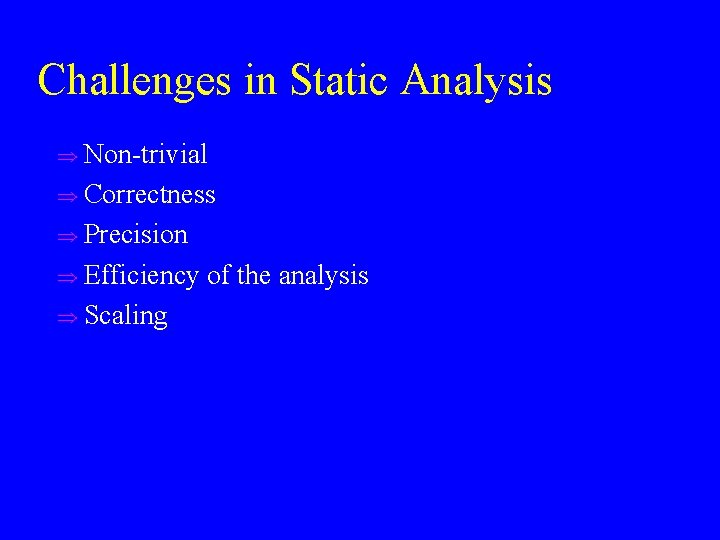Challenges in Static Analysis u Non-trivial u Correctness u Precision u Efficiency u Scaling of the analysisC Compilers u The language was designed to reduce the need for optimizations and static analysis u The programmer has control over performance (order of evaluation, storage, registers( u C compilers nowadays spend most of the compilation time in static analysis u Sometimes C compilers have to work harder!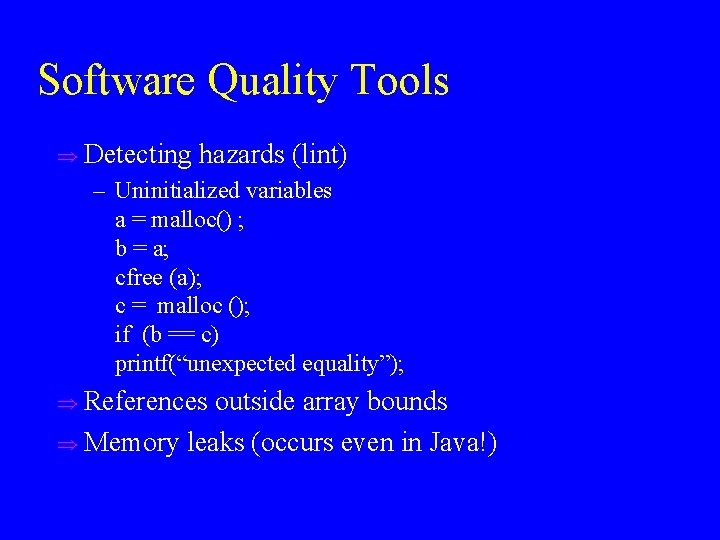Software Quality Tools u Detecting hazards (lint) – Uninitialized variables a = malloc() ; b = a; cfree (a); c = malloc (); if (b == c) printf(“unexpected equality”); u References outside array bounds u Memory leaks (occurs even in Java!)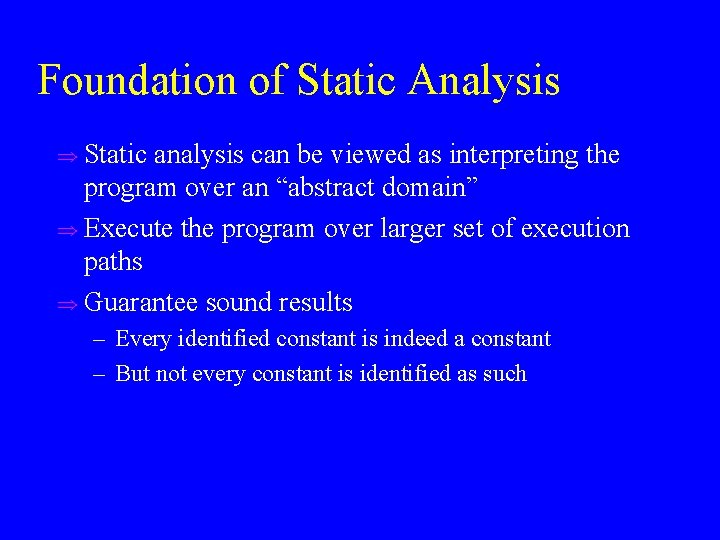Foundation of Static Analysis u Static analysis can be viewed as interpreting the program over an “abstract domain” u Execute the program over larger set of execution paths u Guarantee sound results – Every identified constant is indeed a constant – But not every constant is identified as such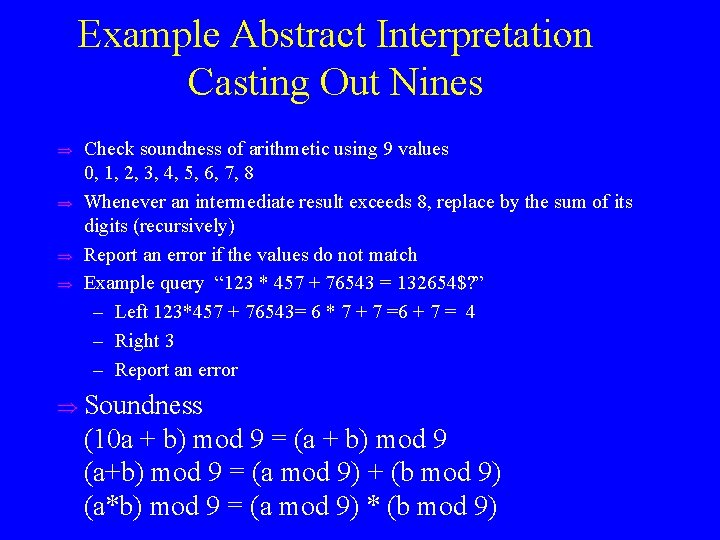Example Abstract Interpretation Casting Out Nines u u Check soundness of arithmetic using 9 values 0, 1, 2, 3, 4, 5, 6, 7, 8 Whenever an intermediate result exceeds 8, replace by the sum of its digits (recursively) Report an error if the values do not match Example query “ 123 * 457 + 76543 = 132654\$? ” – Left 123*457 + 76543= 6 * 7 + 7 =6 + 7 = 4 – Right 3 – Report an error u Soundness (10 a + b) mod 9 = (a + b) mod 9 (a+b) mod 9 = (a mod 9) + (b mod 9) (a*b) mod 9 = (a mod 9) * (b mod 9)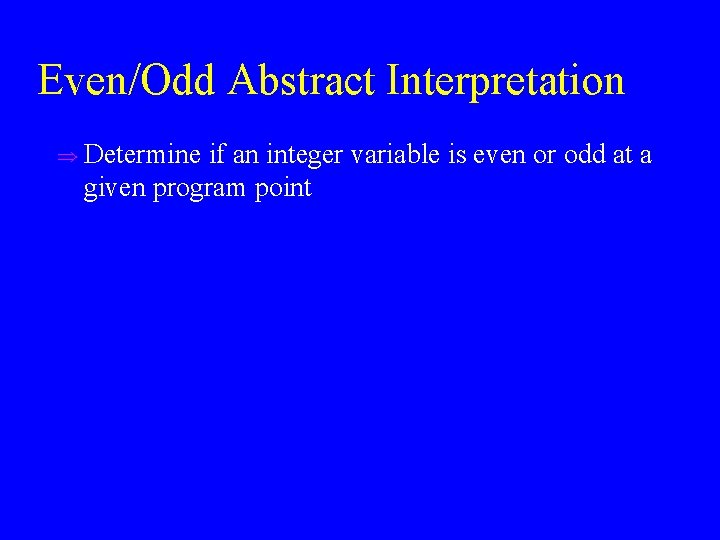Even/Odd Abstract Interpretation u Determine if an integer variable is even or odd at a given program pointExample Program {0, 1, 2, … } while (x !=1) do { {0, 2, 3, … } if (x %2) == 0 { x : = x / 2; } {0, 2, 4, …} {0, 1, 2, … } else { x : = x * 3 + 1; {3, 5, 7, …} {10, 16, 22, …} assert (x %2 ==0); } } {1 }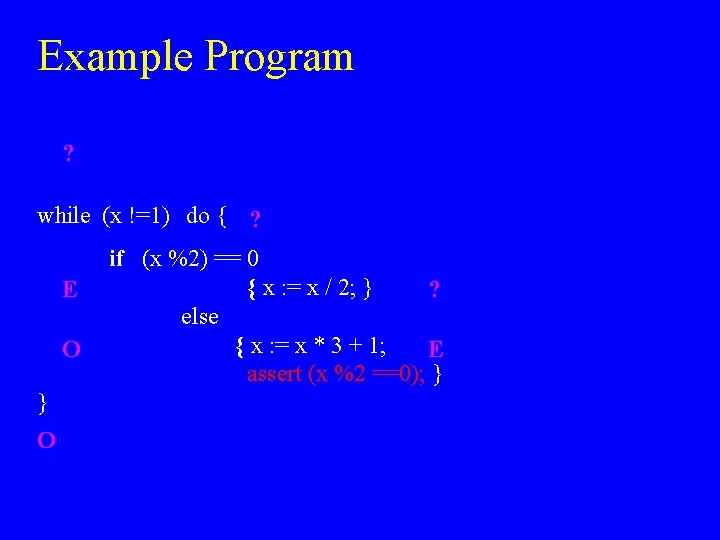Example Program ? while (x !=1) do { ? E O } O if (x %2) == 0 { x : = x / 2; } ? else { x : = x * 3 + 1; E assert (x %2 ==0); }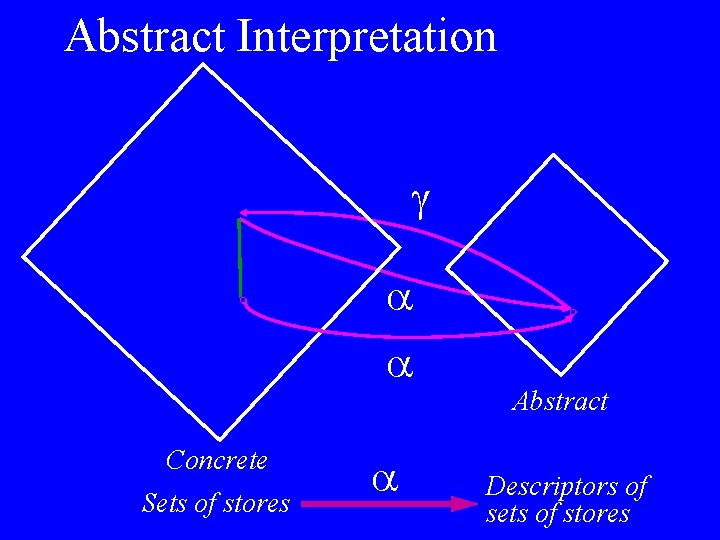Abstract Interpretation Concrete Sets of stores Abstract Descriptors of sets of stores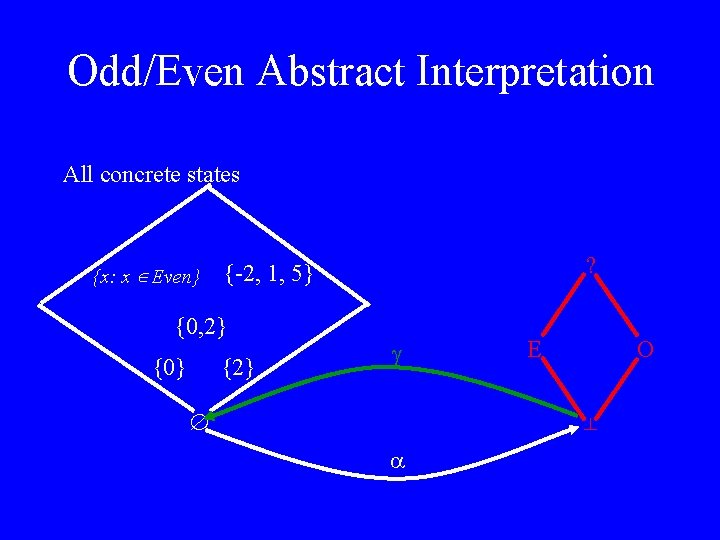Odd/Even Abstract Interpretation All concrete states {x: x Even} {0, 2} {0} ? {-2, 1, 5} {2} E O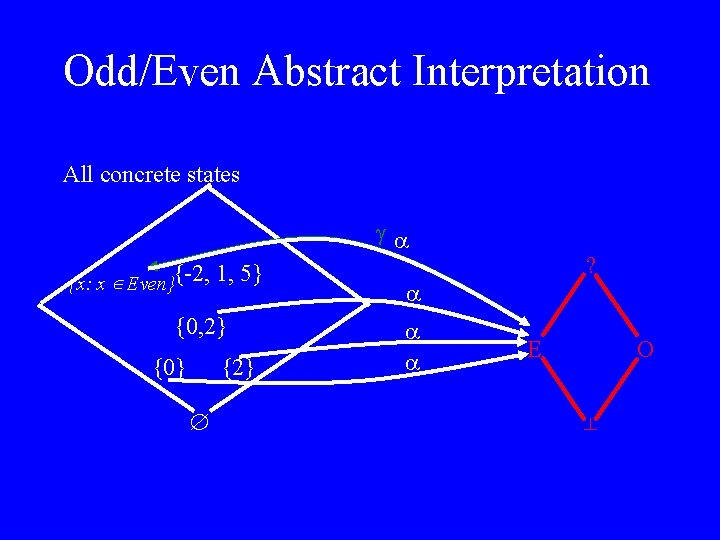Odd/Even Abstract Interpretation All concrete states {x: x Even}{-2, 1, 5} {0, 2} {0} {2} ? E OOdd/Even Abstract Interpretation All concrete states {x: x Even}{-2, 1, 5} {0, 2} {0} {2} ? E O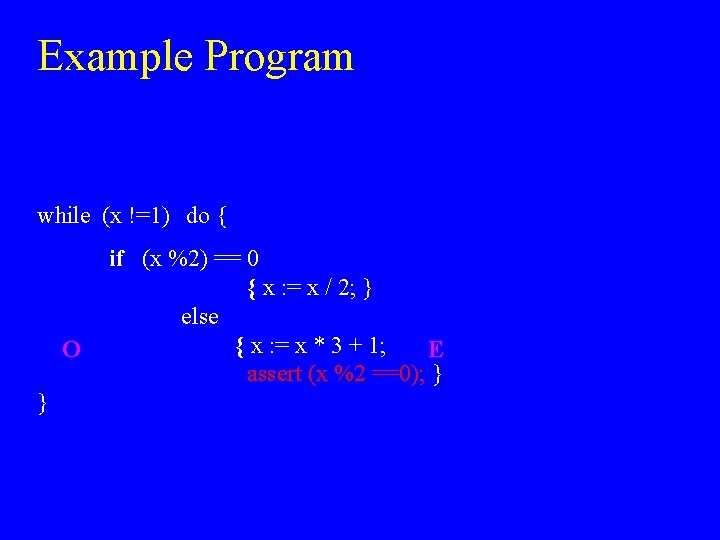Example Program while (x !=1) do { O } if (x %2) == 0 { x : = x / 2; } else { x : = x * 3 + 1; E assert (x %2 ==0); }(Best) Abstract Transformer Operational Semantics Concrete Representation St Concretization Abstract Representation Concrete Representation Abstraction St Abstract Semantics Abstract Representation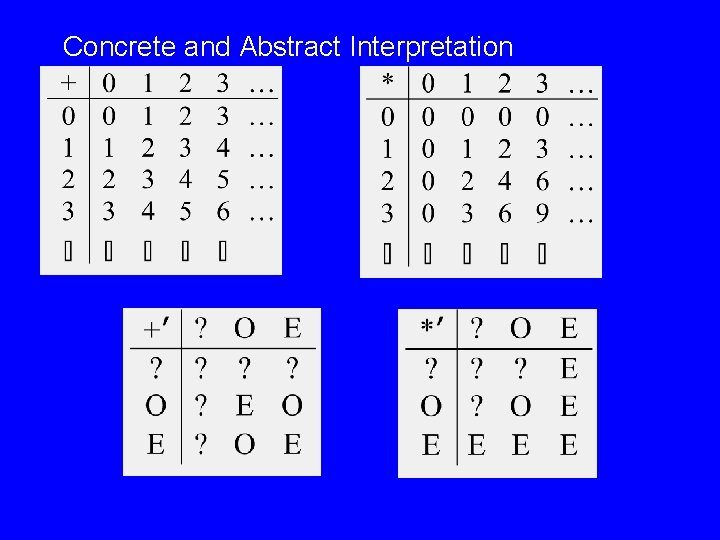Concrete and Abstract Interpretation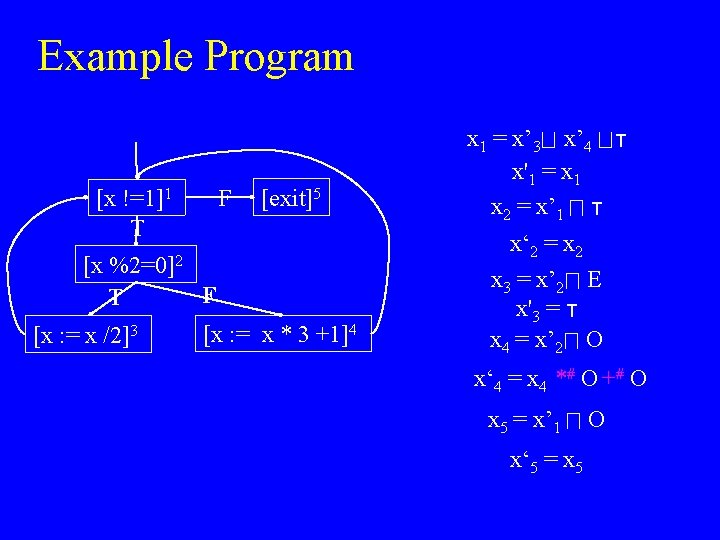Example Program F [x !=1]1 T [exit]5 [x %2=0]2 F T [x : = x /2]3 [x : = x * 3 +1]4 x 1 = x’ 3 x’ 4 x'1 = x 1 x 2 = x’ 1 x‘ 2 = x 2 x 3 = x’ 2 E x'3 = x 4 = x’ 2 O x‘ 4 = x 4 *# O +# O x 5 = x’ 1 O x‘ 5 = x 5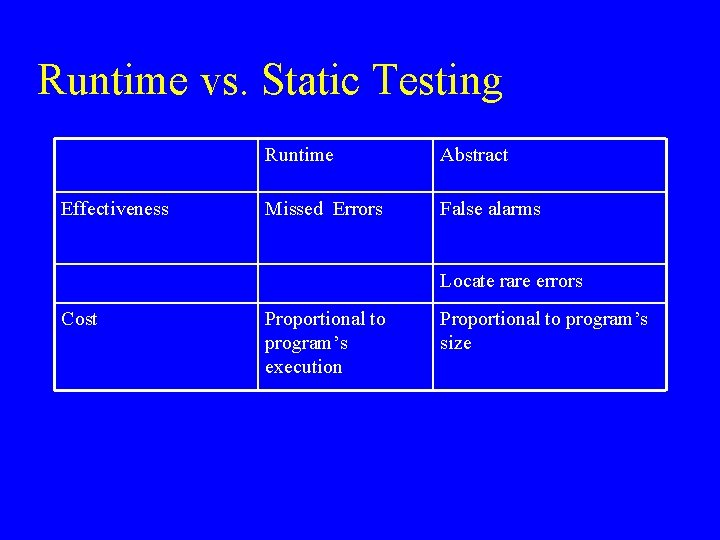Runtime vs. Static Testing Effectiveness Runtime Abstract Missed Errors False alarms Locate rare errors Cost Proportional to program’s execution Proportional to program’s sizeAbstract (Conservative) interpretation Set of states Operational semantics statement s concretization abstract representation statement s Abstract semantics Set of states abstraction abstract representation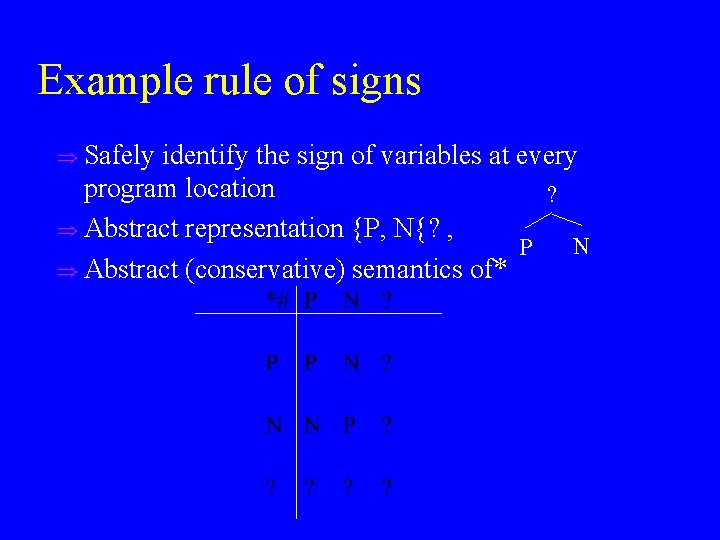Example rule of signs u Safely identify the sign of variables at every program location ? u Abstract representation {P, N{? , N P u Abstract (conservative) semantics of*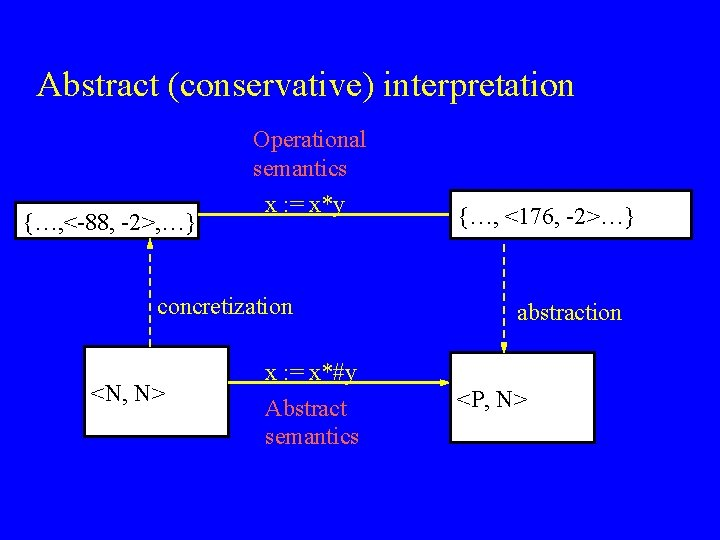Abstract (conservative) interpretation {…, <-88, -2>, …} Operational semantics x : = x*y concretization <N, N> x : = x*#y Abstract semantics {…, <176, -2>…} abstraction <P, N>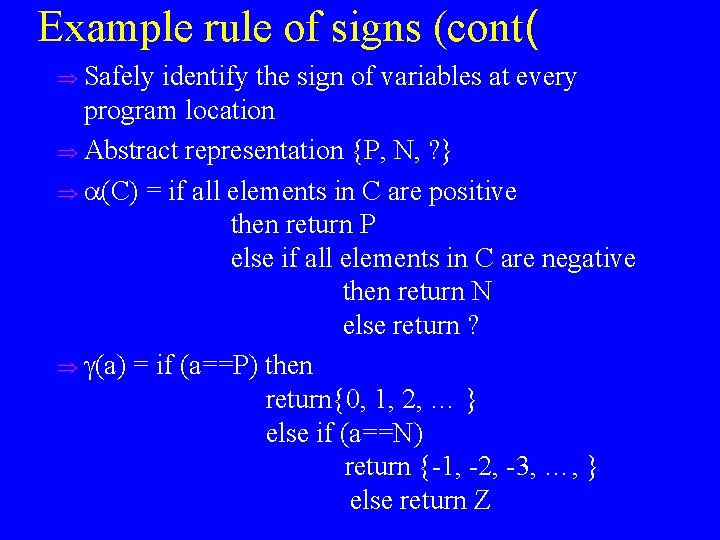Example rule of signs (cont( u Safely identify the sign of variables at every program location u Abstract representation {P, N, ? } u (C) = if all elements in C are positive then return P else if all elements in C are negative then return N else return ? u (a) = if (a==P) then return{0, 1, 2, … } else if (a==N) return {-1, -2, -3, …, } else return Z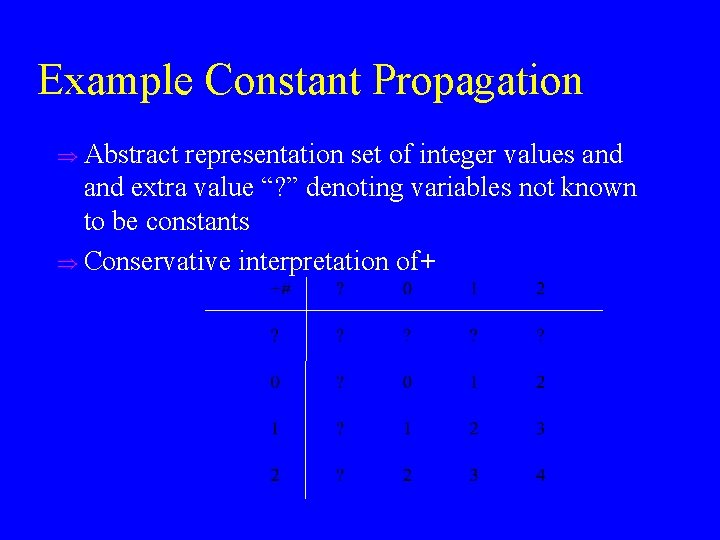Example Constant Propagation u Abstract representation set of integer values and extra value “? ” denoting variables not known to be constants u Conservative interpretation of+Example Constant Propagation(Cont) u Conservative interpretation of*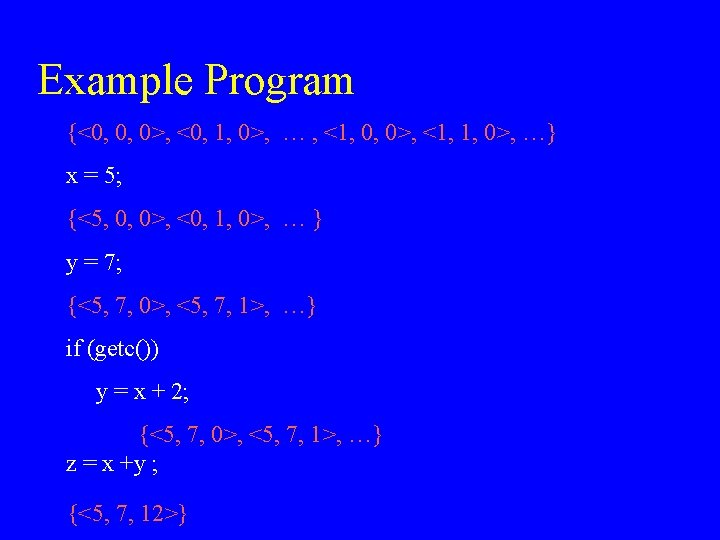Example Program <? , ? > x = 5; <5, ? > y = 7; <5, 7, ? > if (getc()) y = x + 2; <5, 7, ? > z = x +y ; <5, 7, 12>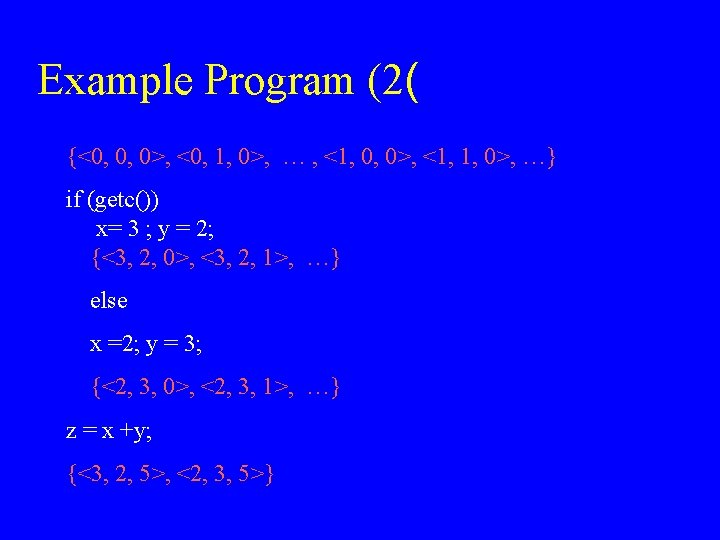Example Program (2( {<0, 0, 0>, <0, 1, 0>, … , <1, 0, 0>, <1, 1, 0>, …} if (getc()) x= 3 ; y = 2; {<3, 2, 0>, <3, 2, 1>, …} else x =2; y = 3; {<2, 3, 0>, <2, 3, 1>, …} z = x +y; {<3, 2, 5>, <2, 3, 5>}Undecidability Issues u It is undecidable if a program point is reachable in some execution u Some static analysis problems are undecidable even if the program conditions are ignored u It may be undecidable to compute the best transformers u Computing the least abstract value may be undecidableThe Constant Propagation Example while (getc()) { if (getc()) x 1 = x 1 + 1; if (getc()) x 2 = x 2 + 1; . . . if (getc()) xn = xn + 1; } y = truncate (1/ (1 + p 2(x 1, x 2, . . . , xn)) /* Is y=0 here? */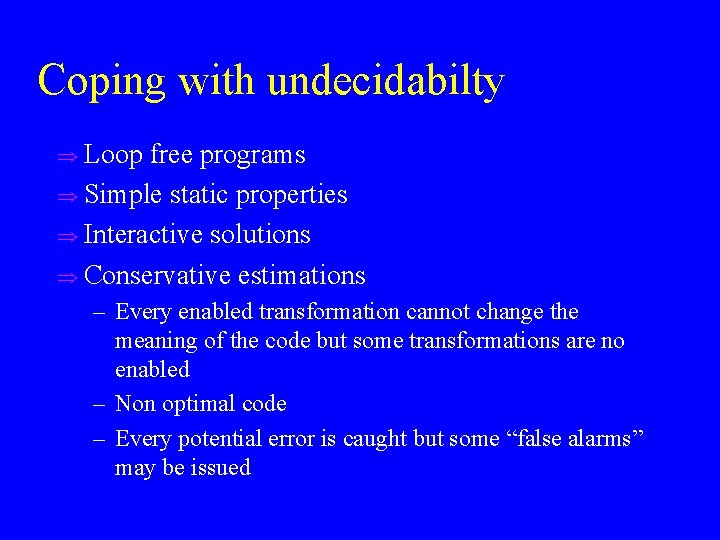Coping with undecidabilty u Loop free programs u Simple static properties u Interactive solutions u Conservative estimations – Every enabled transformation cannot change the meaning of the code but some transformations are no enabled – Non optimal code – Every potential error is caught but some “false alarms” may be issuedAnalogies with Numerical Analysis u Approximate the exact semantics u More precision can be obtained at greater u computational costsViolation of soundness u Loop invariant code motion u Dead code elimination u Overflow ((x+y)+z) != (x + (y+z(( u Quality checking tools may decide to ignore certain kinds of errorsAbstract interpretation cannot be always homomorphic (rules of signs) <-8, 7> Operational semantics x : = x+y <-1, 7> abstraction <N, P> x : = x+#y Abstract semantics abstraction <? P> <N, P>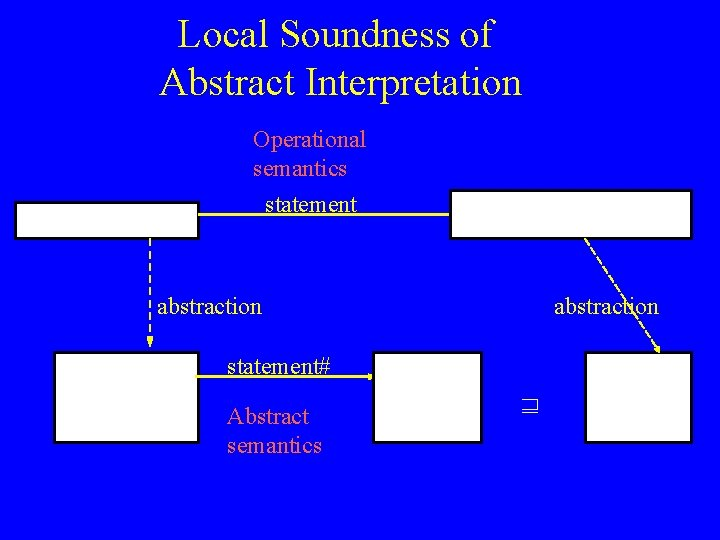Local Soundness of Abstract Interpretation Operational semantics statement abstraction statement# Abstract semanticsOptimality Criteria u Precise (with respect to a subset of the programs( u Precise under the assumption that all paths are executable (statically exact( u Relatively optimal with respect to the chosen abstract domain u Good enough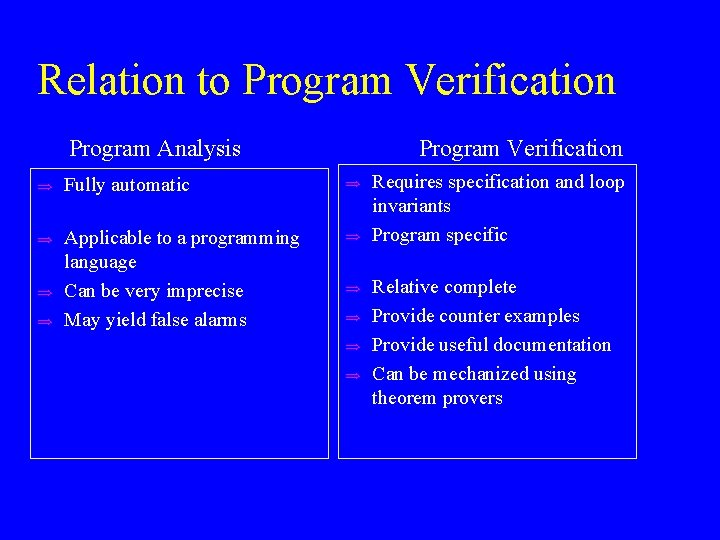Relation to Program Verification Program Analysis Program Verification u Fully automatic u u Applicable to a programming language Can be very imprecise May yield false alarms u u u u Requires specification and loop invariants Program specific Relative complete Provide counter examples Provide useful documentation Can be mechanized using theorem proversOrigins of Abstract Interpretation u u u u [Naur 1965] The Gier Algol compiler “A process which combines the operators and operands of the source text in the manner in which an actual evaluation would have to do it, but which operates on descriptions of the operands, not their value” [Reynolds 1969] Interesting analysis which includes infinite domains (context free grammars) [Syntzoff 1972] Well foudedness of programs and termination [Cousot and Cousot 1976, 77, 79, …] The foundation [Graham and Wegman, 1975: Kamm and Ullman, Kildall 1977] Algorithmic foundations [Tarjan 1981] Reductions to semi-ring problems [Sharir and Pnueli 1981] Foundation of the interprocedural case [Allen, Kennedy, Cock, Jones, Muchnick and Schwartz]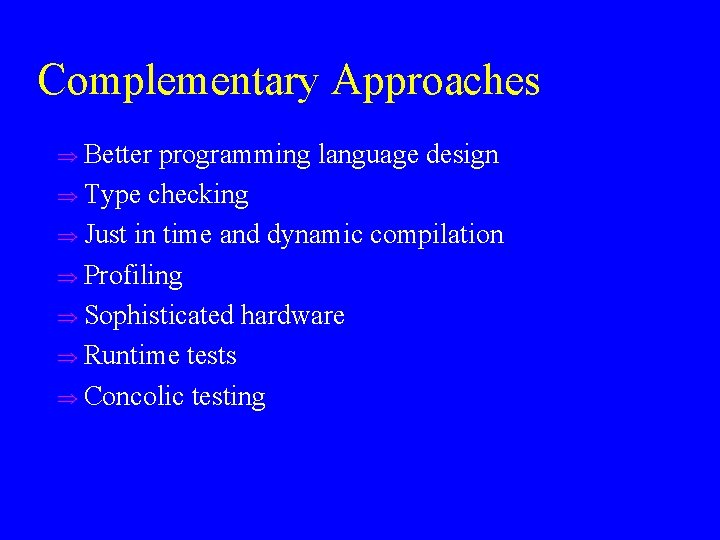Complementary Approaches u Better programming language design u Type checking u Just in time and dynamic compilation u Profiling u Sophisticated hardware u Runtime tests u Concolic testing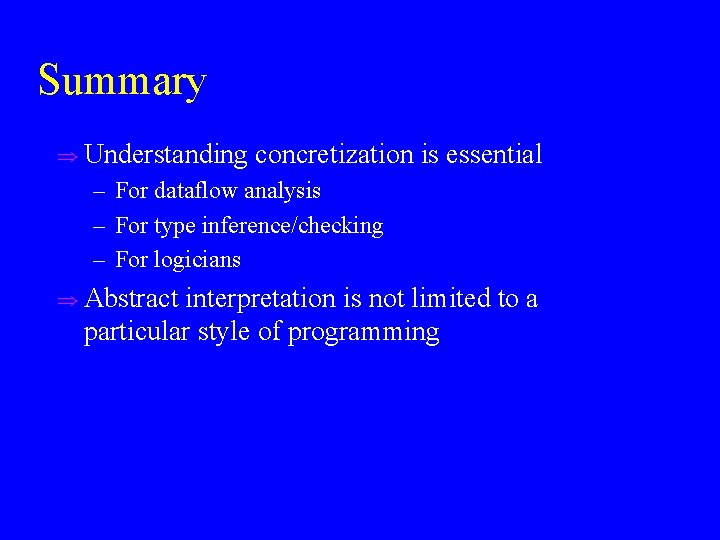Summary u Understanding concretization is essential – For dataflow analysis – For type inference/checking – For logicians u Abstract interpretation is not limited to a particular style of programming